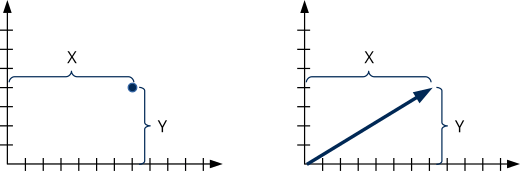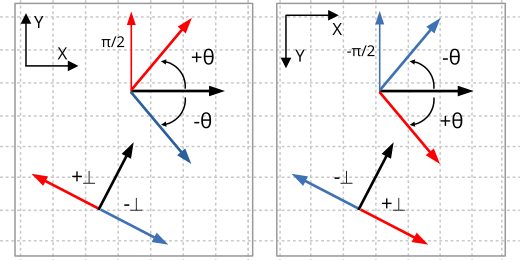# 2D Vectors

This page has been automatically translated using the Google Translate API services. We are working on improving texts. Thank you for your understanding and patience.

## Functions

 V2D v2d (...) V2Df v2d_tof (...) V2Dd v2d_tod (...) V2D v2d_add (...) V2D v2d_sub (...) V2D v2d_mul (...) V2D v2d_from (...) V2D v2d_mid (...) V2D v2d_unit (...) V2D v2d_unit_xy (...) V2D v2d_perp_pos (...) V2D v2d_perp_neg (...) V2D v2d_from_angle (...) bool_t v2d_norm (...) real v2d_length (...) real v2d_sqlength (...) real v2d_dot (...) real v2d_dist (...) real v2d_sqdist (...) real v2d_angle (...) void v2d_rotate (...)

## Types and Constants

Vector (`V2Df`, `V2Dd`) is the most elementary geometric element. It represents a point, a direction or displacement by its two components x and y (Figure 1).Figure 1: A 2D vector represents a position or a displacement in the plane.

The Vectorial Albegra defines a series of basic operations: Addition, negation, multiplication by a scalar, module and normalization (Formula 1). The visual representation of these operations is in (Figure 2).

• Use v2d_subf to subtract two vectors.
• Use v2d_mulf to multiply by a scalar.
• Use v2d_lengthf to calculate the modulus of a vector.
• Use v2d_normf to normalize a vector.

## 1. CW and CCW angles

The angle of rotation of a vector will always be expressed in radians and the positive direction corresponds to the rotation from the X axis to the Y axis. Normally the counterclockwise direction is associated as positive and the clockwise direction negative. This is true in Cartesian coordinates but not in other types of reference systems, such as images or monitors (Figure 3). We must bear this in mind to avoid confusion, something that happens relatively frequently. The same criterion is applied when calculating the perpendicular vector, differentiating between positive and negative.

To avoid confusion, remember that the positive direction is the one that rotates from the X axis to the Y axis. It will be counterclockwise direction in Cartesian coordinates and clockwise direction in screen coordinates.Figure 3: Rotation of a vector in Cartesian and screen systems.

## 2. Vector projection

Another operation used quite frequently in geometry is the projection of points onto a vector. Intuitively, we can see it as the point on the vector closest to the original point and that it will always be on the perpendicular line. We will calculate it with the dot product (Formula 2) and its value (scalar) will be the distance from the origin to the projection in the direction of the vector (Figure 4).

• Use v2d_dotf to calculate the dot product of two vectors.
If we are interested in the relative position between different projections, we can avoid dividing by the vector's modulus, which is more computationally efficient by not calculating square roots.

## kZERO

```const V2Df kV2D_ZEROf;

const V2Dd kV2D_ZEROd;

const V2D V2D::kZERO;```

The (0,0) vector.

## kX

```const V2Df kV2D_Xf;

const V2Dd kV2D_Xd;

const V2D V2D::kX;```

The (1,0) vector.

## kY

```const V2Df kV2D_Yf;

const V2Dd kV2D_Yd;

const V2D V2D::kY;```

The (,1) vector.

## v2d ()

Create a 2d vector from its components.

```V2Df
v2df(const real32_t x,
const real32_t y);

V2Dd
v2dd(const real64_t x,
const real64_t y);

V2D
V2D(const real x,
const real y);
```
 x X coordinate. y Y coordinate.

Return

2d vector.

## v2d_tof ()

Convert a vector from double to float.

```V2Df
v2d_tof(const V2Dd *v);
```
 v Vector.

Return

The 2d vector in simple precision.

## v2d_tod ()

Convert a vector from float to double.

```V2Dd
v2d_tod(const V2Df *v);
```
 v Vector.

Return

The 2d vector in double precision.

```V2Df
const V2Df *v2);

V2Dd
const V2Dd *v2);

V2D
const V2D *v2);
```
 v1 Vector 1. v2 Vector 2.

Return

The result vector.

## v2d_sub ()

Subtract two vectors.

```V2Df
v2d_subf(const V2Df *v1,
const V2Df *v2);

V2Dd
v2d_subd(const V2Dd *v1,
const V2Dd *v2);

V2D
V2D::sub(const V2D *v1,
const V2D *v2);
```
 v1 Vector 1. v2 Vector 2.

Return

The result vector.

## v2d_mul ()

Multiply a vector by a scalar.

```V2Df
v2d_mulf(const V2Df *v,
const real32_t s);

V2Dd
v2d_muld(const V2Dd *v,
const real64_t s);

V2D
V2D::mul(const V2D *v,
const real s);
```
 v Vector. s Scalar.

Return

The result vector.

## v2d_from ()

Create a vector from a point and a direction.

```V2Df
v2d_fromf(const V2Df *v,
const V2Df *dir,
const real32_t length);

V2Dd
v2d_fromd(const V2Dd *v,
const V2Dd *dir,
const real64_t length);

V2D
V2D::from(const V2D *v,
const V2D *dir,
const real length);
```
 v Initial vector. dir Direction. length Length.

Return

The result vector.

Remarks

It will perform the operation `r = v + length * dir`. `dir` does not need to be unitary, in which case `length` will behave as a scale factor.

## v2d_mid ()

Returns the midpoint of two points.

```V2Df
v2d_midf(const V2Df *v1,
const V2Df *v2);

V2Dd
v2d_midd(const V2Dd *v1,
const V2Dd *v2);

V2D
V2D::mid(const V2D *v1,
const V2D *v2);
```
 v1 First point. v2 Second point.

Return

The middle point.

## v2d_unit ()

Unit vector (direction) from 1 to 2.

```V2Df
v2d_unitf(const V2Df *v1,
const V2Df *v2,
real32_t *dist);

V2Dd
v2d_unitd(const V2Dd *v1,
const V2Dd *v2,
real64_t *dist);

V2D
V2D::unit(const V2D *v1,
const V2D *v2,
real *dist);
```
 v1 Point 1 (origin). v2 Point 2 (destination). dist Distance between points. Can be `NULL`.

Return

The unit vector.

## v2d_unit_xy ()

Unit vector (direction) from 1 to 2.

```V2Df
v2d_unit_xyf(const real32_t x1,
const real32_t y1,
const real32_t x2,
const real32_t y2,
real32_t *dist);

V2Dd
v2d_unit_xyd(const real64_t x1,
const real64_t y1,
const real64_t x2,
const real64_t y2,
real64_t *dist);

V2D
V2D::unit_xy(const real x1,
const real y1,
const real x2,
const real y2,
real *dist);
```
 x1 X coordinate of point 1 (origin). y1 Y coordinate of point 1 (origin). x2 X coordinate of point 2 (destination). y2 Y coordinate of point 2 (destination). dist Distance between points. Can be `NULL`.

Return

The unit vector.

## v2d_perp_pos ()

Gets the positive perpendicular vector.

```V2Df
v2d_perp_posf(const V2Df *v);

V2Dd
v2d_perp_posd(const V2Dd *v);

V2D
V2D::perp_pos(const V2D *v);
```
 v Initial vector.

Return

The perpendicular vector.

Remarks

It is the perpendicular obtained by positive angle (+π/2).

## v2d_perp_neg ()

Gets the negative perpendicular vector.

```V2Df
v2d_perp_negf(const V2Df *v);

V2Dd
v2d_perp_negd(const V2Dd *v);

V2D
V2D::perp_neg(const V2D *v);
```
 v Initial vector.

Return

The perpendicular vector.

Remarks

It is the perpendicular obtained by negative angle (-π/2).

## v2d_from_angle ()

Gets the vector resulting from applying a rotation to the vector `[1,0]`.

```V2Df
v2d_from_anglef(const real32_t a);

V2Dd
v2d_from_angled(const real64_t a);

V2D
V2D::from_angle(const real a);
```
 a Angle.

Return

The vector.

Remarks

For `a=0` we get `[1,0]`. For `a=π/2` `[0,1]`.

## v2d_norm ()

Normalize a vector, that is, make it a vector of length = 1.

```bool_t
v2d_normf(V2Df *v);

bool_t
v2d_normd(V2Dd *v);

bool_t
V2D::norm(V2D *v);
```
 v Vector that will be normalized.

Return

`FALSE` if the vector cannot be normalized (vector 0).

## v2d_length ()

Calculate the length of a vector.

```real32_t
v2d_lengthf(const V2Df *v);

real64_t
v2d_lengthd(const V2Dd *v);

real
V2D::length(const V2D *v);
```
 v Vector.

Return

The vector module.

## v2d_sqlength ()

Calculate the square of the length of a vector.

```real32_t
v2d_sqlengthf(const V2Df *v);

real64_t
v2d_sqlengthd(const V2Dd *v);

real
V2D::sqlength(const V2D *v);
```
 v Vector.

Return

The square of the vector modulus.

Remarks

Avoid using the square root, so it is more efficient than v2d_lengthf. Often used to compare distances.

## v2d_dot ()

Product of two vectors.

```real32_t
v2d_dotf(const V2Df *v1,
const V2Df *v2);

real64_t
v2d_dotd(const V2Dd *v1,
const V2Dd *v2);

real
V2D::dot(const V2D *v1,
const V2D *v2);
```
 v1 Vector 1. v2 Vector 2.

Return

Scalar product.

## v2d_dist ()

Calculate the distance between two points.

```real32_t
v2d_distf(const V2Df *v1,
const V2Df *v2);

real64_t
v2d_distd(const V2Dd *v1,
const V2Dd *v2);

real
V2D::dist(const V2D *v1,
const V2D *v2);
```
 v1 The first point. v2 The second point.

Return

Distance.

## v2d_sqdist ()

Calculate the square of the distance between two points.

```real32_t
v2d_sqdistf(const V2Df *v1,
const V2Df *v2);

real64_t
v2d_sqdistd(const V2Dd *v1,
const V2Dd *v2);

real
V2D::sqdist(const V2D *v1,
const V2D *v2);
```
 v1 The first point. v2 The second point.

Return

The distance squared.

Remarks

It avoids using the square root, so it is more efficient than v2d_distf. Often used to compare distances.

## v2d_angle ()

Calculate the angle formed by two vectors.

```real32_t
v2d_anglef(const V2Df *v1,
const V2Df *v2);

real64_t
v2d_angled(const V2Dd *v1,
const V2Dd *v2);

real
V2D::angle(const V2D *v1,
const V2D *v2);
```
 v1 Vector 1. v2 Vector 2.

Return

The angle in radians (-Pi, Pi)

Remarks

Positive angles go from v1 to v2 counterclockwise. For angles greater than Pi radians (180°) it will return negative (clockwise).

## v2d_rotate ()

Apply a rotation to a vector.

```void
v2d_rotatef(V2Df *v,
const real32_t a);

void
v2d_rotated(V2Dd *v,
const real64_t a);

void
V2D::rotate(V2D *v,
const real a);
```
 v Vector to be rotated (origin/destination. a Angle in radians.

Remarks

This function involves calculating the sine and cosine. Use t2d_vmultnf if you have to apply the same rotation to multiple vectors.